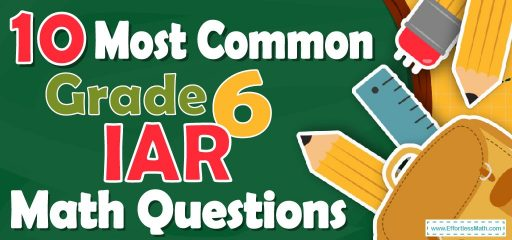# 10 Most Common 6th Grade IAR Math QuestionsBecause 6th-grade students’ scores on the Illinois Assessment of Readiness (IAR) math test affect their fate, we also decided to compile 10 Most Common 6th Grade IAR Math Questions for 6th-Grade IAR Math and provide them to 6th-grade students.

These questions are very similar to the main test questions and this helps a lot in 6th-grade students mastering the concepts of the 6th-grade IAR Math Test.

Make sure to follow some of the related links at the bottom of this post to get a better idea of what kind of mathematics questions your students need to practice.

The Absolute Best Book to Ace the IAR Math Test

## 10 Sample 6th Grade IAR Math Practice Questions

1- What is the equation of a line that passes through points $$(0, 4)$$ and $$(2, 8)$$?

A. $$y = x$$

B. $$y = x + 4$$

C. $$y = 2x + 4$$

D. $$y = 2x – 4$$

2- What is the volume of a box with the following dimensions?

Height $$= 6 cm$$ , Width $$= 7 cm$$, Length $$= 9 cm$$

A. $$63 \space cm^3$$

B. $$126\space cm^3$$

C. $$189\space cm^3$$

D. $$378\space cm^3$$

3- Anita’s trick–or–treat bag contains $$14$$ pieces of chocolate, $$15$$ suckers, $$16$$ pieces of gum, and $$20$$ pieces of licorice. If she randomly pulls a piece of candy from her bag, what is the probability of her pulling out a piece of sucker?

A. $$\frac{1}{13}$$

B. $$\frac{3}{13}$$

C. $$\frac{14}{65}$$

D. $$\frac{16}{65}$$

4-

In the following rectangle, which statement is false?

A. AD is parallel to BC

B. The measure of the sum of all the angles equals $$360^\circ$$.

C. Length of AB equal to length DC.

D. AB is perpendicular to AC.

5- The area of a rectangular yard is $$90$$ square meters. What is its width if its length is $$15$$ meters?

A. $$10$$ meters

B. $$8$$ meters

C. $$6$$ meters

D. $$4$$ meters

6- Which statement about $$4$$ multiplied by $$\frac{3}{5}$$ must be true?

A. The product is between $$1$$ and $$2$$

B. The product is greater than $$3$$

C. The product is equal to $$\frac{75}{31}$$

D. The product is between $$2$$ and $$2.5$$

7- Which of the following lists shows the fractions in order from least to greatest?
$$\frac{3}{4}, \frac{2}{7}, \frac{3}{8} , \frac{5}{11}$$

A. $$\frac{3}{8}, \frac{2}{7}, \frac{3}{4}, \frac{5}{11}$$

B. $$\frac{2}{7}, \frac{5}{11}, \frac{3}{8}, \frac{3}{4}$$

C. $$\frac{2}{7}, \frac{3}{8}, \frac{5}{11}, \frac{3}{4}$$

D. $$\frac{3}{8}, \frac{2}{7}, \frac{5}{11}, \frac{3}{4}$$

8- A car costing $$300$$ is discounted $$10\%$$. Which of the following expressions can be used to find the selling price of the car?

A. $$(300)(0.4)$$

B. $$300-(300×0.1)$$

C. $$(300)(0.1)$$

D. $$300-(300×0.9)$$

9- What is the missing price factor of the number $$420$$?
$$420=2^2×3^1×…$$

A. $$2^2×3^1×5^1×7^1$$

B. $$2^2×3^1×7^1×9^1$$

C. $$1^2×2^3×2^1×3^1$$

D. $$3^2×5^1×7^1×9^1$$

10- If the area of the following trapezoid is equal to $$A$$, which equation represents $$x$$?

A. $$x = \frac{13}{A}$$

B. $$x = \frac{A}{13}$$

C. $$x=A+13$$

D. $$x=A-13$$

Best 6th Grade IAR Math Practice Resource

1- C
The slope of the line is:
$$\frac{y_2-y_1}{x_2-x_1 }= \frac{8 – 4}{2 – 0}=\frac{4}{2}=2$$
The equation of a line can be written as:
$$y-y_0=m(x – x_0 )→y-4 = 2(x – 0)→y-4 = 2x→y = 2x + 4$$

2- D
Volume of a $$box = length × width × height = 6 × 7 × 9 = 378$$

3- B
$$Probability = \frac{number \space of \space desired \space outcomes}{number \space of \space total \space outcomes}=\frac{15}{14+15+16+20}= \frac{15}{65}=\frac{3}{13}$$

4- D
In any rectangle, sides are not perpendicular to diagonals.

5- C
Let $$y$$ be the width of the rectangle. Then; $$15×y=90→y=\frac{90}{15}=6$$

6- D
$$4×\frac{3}{5}=\frac{12}{5}=2.4$$
$$2.4>2$$
$$2.4<3$$
$$\frac{75}{31}=2.419≠2.4$$
$$2<2.4<2.5$$ This is the answer!

7- C
Let’s compare each fraction:
$$\frac{2}{7}< \frac{3}{8}< \frac{5}{11} < \frac{3}{4}$$
Only choice C provides the right order.

8- B
To find the discount, multiply the number ($$100\%$$$$-$$ rate of discount)
Therefore; $$300(100\%-10\%)=300(1-0.1)=300-(300×0.1)$$

9- A
$$420=2^2×3^1×5^1×7^1$$

10- B

The area of the trapezoid is: area= $$\frac{(base 1+base 2)}{2}×height= (\frac{10 + 16}{2})x = A$$
$$→13x = A→x = \frac{A}{13}$$

The Most Comprehensive Review for 6th-Grade Students

### What people say about "10 Most Common 6th Grade IAR Math Questions - Effortless Math: We Help Students Learn to LOVE Mathematics"?

No one replied yet.

X
51% OFF

Limited time only!

Save Over 51%

SAVE $15 It was$29.99 now it is \$14.99